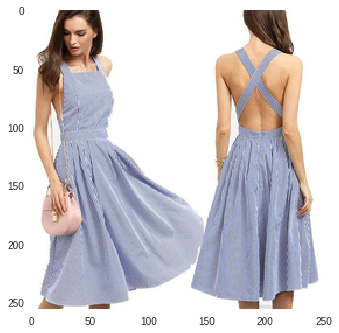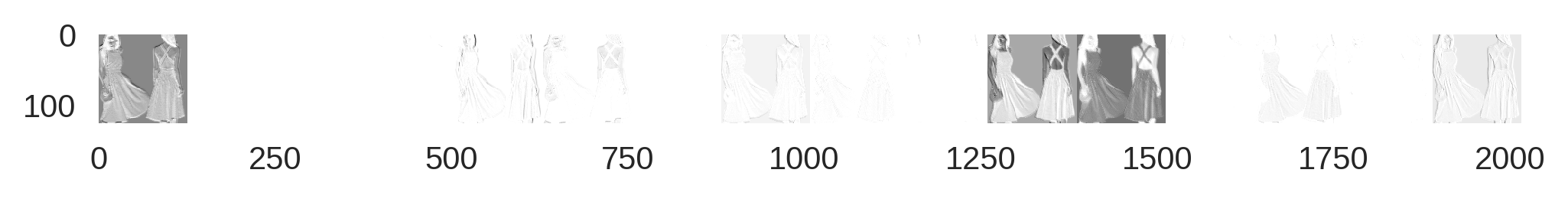### Learning About Fashion With A Convolutional Neural NetworkSo for the past week or so I have been working on a model, a convolutional neural network to participate in a kaggle competition. I have been developing it on google’s colab project. See my last post to get an idea about what google colab is all about. Anyway, this is a fashion style prediction competition. Now, I’m not that into fashion, but I have been looking for an image recognition challenge project that I could share with you guys for a while, and try out a convolutional neural network. It has been kind of fun to mess around a little bit, and try to get things working.

I stored the data on google drive in its raw json format so I needed to link up to that in order to feed data into an algorithm. I think that this would require a bit of explaining, so we’ll ignore the overhead. Especially, since a lot of my code cells on github are just me experimenting with google colab, or just messing around trying to get keras to give me something interesting to work with. Perhaps in a later post I will go into how some of these things work. For now, I will just go over the code for my convolutional neural network that I am training through colab’s gpu.

Today, I just want to go over the image classification problem itself, and the code that I have in order to classify these images using a convolutional neural network. A lot of my code is actually designed to persist the model. It takes about an hour to run one epoch of the neural network, and you only get 12 hours at a time. I run it for 5 epochs at a time, because I’m paranoid about the whole thing falling down. Plus I have it saving at a couple of points.

### Onto the Convolutional Neural Network

Convolutional neural networks get their names because we are learning a bunch of convolutional layers. I’m going to explain a convolution like this:

Convolutions are a specific way to pass one functions or input through another. You can think of a convolution as a filter. Yp pass an input through the filter and out pops the same data, just filtered down in some way. For example in image data, you might think that it would be useful to know where the edges in the image are located. That is where a convolutional neural net really shines. It will learn how to detect the edges of an image all by itself. Each layer in a convolutional neural network is learning a set of filters, like how to detect the edges in an image, or to strip out all of the blue from an image.

So the biggest pain was just grabbing the data in such a way that it doesn’t fill my hard drive, and so that it won’t be painfully slow to train the network because of the latency feeding it data. So the first thing that I needed to do was to get the data. I downloaded the raw data from the Kaggle competition in its json format to google drive. And then to conserve space, I decided not to save the images anywhere. Rather, I decided that I would just ping the server for a picture when I needed it. So I stored the urls, and just used those to get what I needed when I needed it.

import json
print('okay')
print('cool')
print('done')
print('removed extraneous data')
urls = [obj['url'] for obj in data['images']]


Great now that I’ve got the data, I need to get the images off of the internet and feed them to my model. That’s why we’re going to build a nice little generator to do that. This generator supports multithreading, so it was fun to write and debug. One thing that I want to mention about this generator is that I am pulling images randomly, 32 at a time. The reason is that I need a batch and that seemed like a reasonable number for a batch. The reason that it is random is that I wanted it to be fault tolerant. This way, if I have to restart for any reason, I won’t retrain on the same images from beginning to end.

from keras.preprocessing.image import ImageDataGenerator
from itertools import chain, repeat, cycle
import pandas as pd
import numpy as np
from PIL import Image
import matplotlib.pyplot as plt
import os
from urllib.request import urlopen

TARGET_SIZE = (256, 256)
BATCH_SIZE = 128

print('meh')
output = []
for dat in data['annotations']:
temp = *228
for obj in dat['labelId']:
temp[int(obj)-1] = 1
output.append(temp)
depvar = np.array(output)

print('mmmmmm donuts')
class BatchGenerator:

def __init__(self, batch_size=32,target_size=(256,256)):
self.batch_size = batch_size
self.TARGET_SIZE=target_size

def __iter__(self):
return self

def __next__(self):
with self.lock:
while True:
X=[]
y=[]
for i in range(32):
pic = np.random.randint(0,len(urls))
tempnames = depvar[pic].reshape(1,228)
try:
img_file = urlopen(urls[pic])
im = Image.open(img_file)
except:
output = *(256*256*3)
output = np.array(output).reshape(256,256,3).astype('uint8')
im = Image.fromarray(output).convert('RGB')
im2 = im.resize(self.TARGET_SIZE, Image.ANTIALIAS)
im2.thumbnail(self.TARGET_SIZE, Image.ANTIALIAS)
#plt.imshow(np.asarray(im2))
#plt.show()

X_batch = np.asarray(im2).reshape(1,256,256,3)
names = depvar[pic]
#                if np.random.rand()>1:
#                    zoomed = np.asarray(im2.resize((512,512), Image.ANTIALIAS))
#                    choice = np.random.randint(0,256)
#                    #choice = 128
#                    X_batch = zoomed[choice:choice+256,choice:choice+256,:].reshape(1,256,256,3)
#                if np.random.rand()>1:
#                    X_batch = X_batch[:,::-1,:,:]
names.reshape(1,228)
X_batch = X_batch/255.0
X.append(X_batch)
y.append(names)
return np.array(X).reshape(32,256,256,3), np.array(y).reshape(32,228)
def next(self):
return self.__next__()

print('check')

train_gen = BatchGenerator(batch_size=1631)
val_gen = BatchGenerator()
#print(train_gen.next())


Yeah, I know that was a ton of code, but bear with me. We’re almost to the neural network portion. I need one more helper function, actually, I don’t but it is a holdover from a previous version that I put together. The previous version is also on github.

from urllib.request import urlopen
from PIL import Image
def convert_pic_to_array(url):
size = (256, 256)
try:
print('pullimg')
img_file = urlopen(url)
print('imgopen')
im = Image.open(img_file)
print('gothere')
except:
output = *(256*256*3)
output = np.array(output).reshape(1,256,256,3)
return(output)

im2 = im.resize(size, Image.ANTIALIAS)
im2.thumbnail(size, Image.ANTIALIAS)
output = np.asarray(im2)
#print(conv_base.predict(output.reshape(1,256,256,3)).shape)
return(output.reshape(1,256,256,3))
convert_pic_to_array(urls)
urlopen(urls)


Okay, so now that we got everything together, we can start talking about defining the neural network that we’ll be using. After experimenting a lot about what would and wouldn’t crash google colab, I settled in on the deepest network that I could in order to classify these images. You can see from the code that I’m going to share that I started with something a little ambitious. Actually, I played with a network that was only two layers deep to see if I could get that to work in a very sad, yet, super fast to converge, it won’t get any better than this sort of way. And to make sure that it was going to do what I wanted it to do. Once I got that to work, I’ll talk about key take aways towards the end of this post, I started my ambitious “gigantic” (relatively speaking) network. I paired it down until it worked.

from keras.models import Sequential
from keras.layers import Dense, Dropout
from keras.layers import Reshape
from keras.layers.core import Activation
from keras.layers.normalization import BatchNormalization
from keras.layers.convolutional import UpSampling2D
from keras.layers.convolutional import Conv2D, MaxPooling2D
from keras.layers.core import Flatten
from keras.preprocessing.image import ImageDataGenerator
from PIL import Image
#from keras.applications import VGG19

def discriminator_model():

model = Sequential()
(6, 6),
strides=(2,2),
input_shape=( 256, 256, 3),
kernel_initializer='glorot_normal',
bias_initializer='zeros',
activation='relu'))

return model
d = discriminator_model()
d.compile(loss='categorical_crossentropy', optimizer=d_optim)


Okay, there it is a deep convolutional network. It is 12 layers deep, ignoring batch normalization, dropout, activations, and max pooling. Yep, a deep network, by the standards of 2011. Today, this network is laughably shallow. But hey, it seems to be getting the job done.

Now that we have our model in place and some way to access the data, we should just get down to the business of fitting the model.

from keras.optimizers import RMSprop, Adam
#from keras.callbacks import ModelCheckpoint
d.compile(loss='categorical_crossentropy', optimizer=d_optim)
#d_optim = RMSprop(lr=0.00001, clipnorm=0.5)
#d.compile(loss='categorical_crossentropy', optimizer=d_optim)
#cweights = {}
#cw = 1/(np.sum(depvar,axis=0)/np.sum(depvar))
#for i in range(len(depvar)):
#    cweights[i] = cw[i]

train_gen = BatchGenerator(batch_size=32)
#for i in range(1000):
x,y = next(train_gen)
from keras.callbacks import ModelCheckpoint
checkpoint = ModelCheckpoint('drive/model_weights.h5')
callbacks_list = [checkpoint]
#if i%4==0:
d.save_weights('drive/model_weights.h5')
history = d.fit_generator(train_gen,steps_per_epoch=2000,epochs=110,callbacks=callbacks_list,workers=7,use_multiprocessing=True)#, validation_data=val_gen,validation_steps=1)
#else:
#d.fit(x,y,epochs=10)
d.save('drive/model.h5')
d.save_weights('drive/model_weights.h5')
test = d.predict(convert_pic_to_array(urls))
li = {}
for i in range(len(test)):
li[i+1]=test[0,i]
print(sorted(li.items(), key=lambda x: x, reverse=True))
print(data['annotations']['labelId'])


Now you may be wondering how the network performs. At the time of writing, not super great. The thing is that training is going really really slowly even on a GPU. Now don’t get me wrong, a batch runs pretty quickly. However, that isn’t the main problem. The problem is getting the data, that’s why I’ve got multiple threads running. I’m using 7 because anything more seems to crash colab. Oh well. Even so, the loss is going down at a painfully slow rate even if I got to use more threads.

### Choices I had to Make For Hyper-Parameters

The learning rate is really small. What I noticed was anything smaller, and the network probably would start creeping down after a billion years of training, anything larger and the loss actually goes up. UP! So training the network is slow. Which also probably has to do with the gradient clipping that I am doing as well. Again it just starts going bananas after a little bit if that’s not in there.

So, I’m going to continue training the network until it gets to where I want it to go. Hopefully, it wil be ready for showtime before the contest ends, but hey, you never know. It might take 6.5 million years to get there, but we’ll get there eventually. And at that time, I will report my results, final oss, and validation loss, and so forth.

### Parting Goodies

I thought that I’d share the activations from the networks first layer (so far). I think it becomes very apparent that we are applying various filters to the data. Here are the filtered outputs from a random image.Filters from partially trained neural net.

And if for whatever reason you think that I am being dishonest about the neural net learning these filters for its first layer, then check out the image that was passed through the network.Original image fed into neural network.